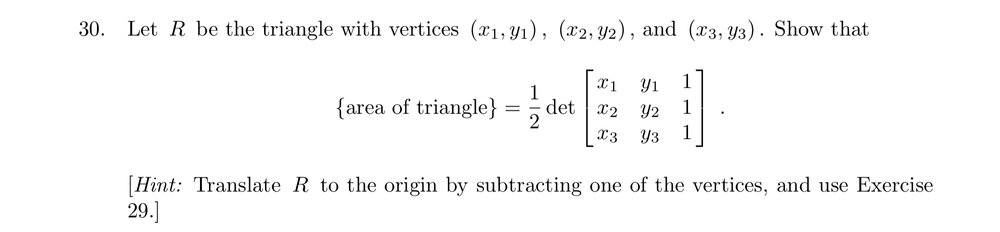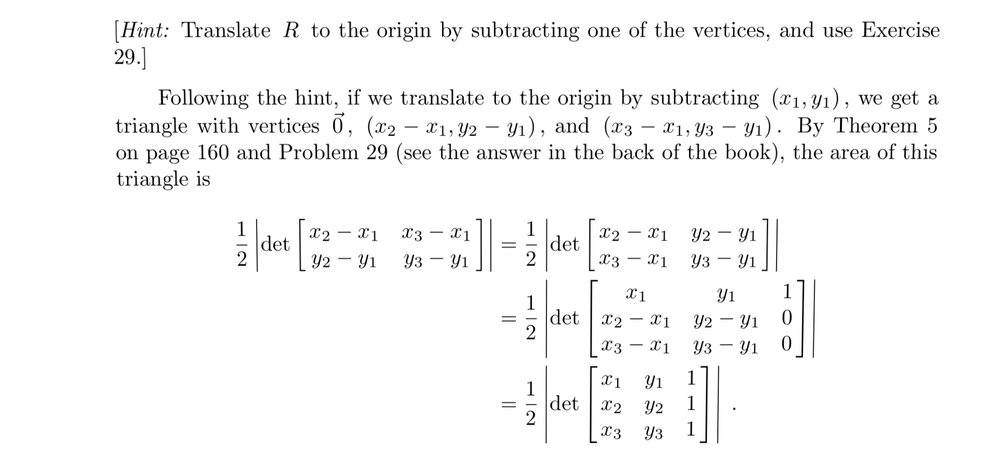# Using a determinant to find the area of the triangle (deriving the formula)

Sunwoo Bae
Homework Statement:
Shown in the text
Relevant Equations:
Det(A) = det(A^T) determinant of transpose equals determinant of the original matrixThis is the question. The following is the solutions I found:I understand that the first line was derived by setting one vertex on origin and taking the transpose of the matrix. However, I cannot understand where the extra row and column came from in the second line. Can anyone explain how the formula is derived?

Thank you!

$$\det \begin{pmatrix}a & b & 1 \\ c & d & 0 \\ e & f & 0\end{pmatrix}= \det \begin{pmatrix} c & d \\ e & f\end{pmatrix}$$
•